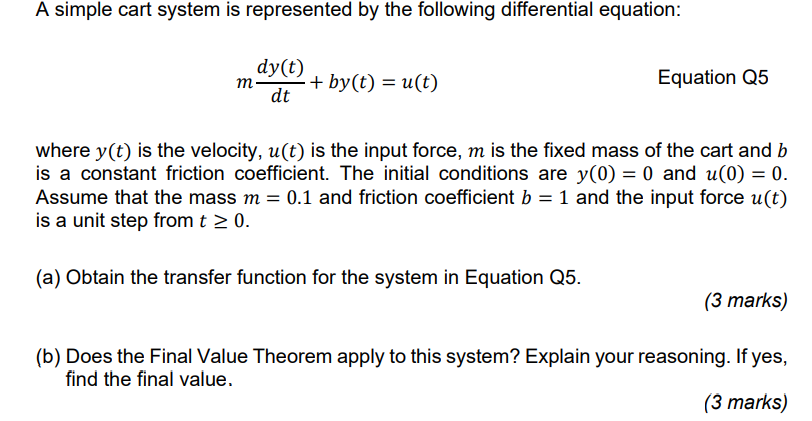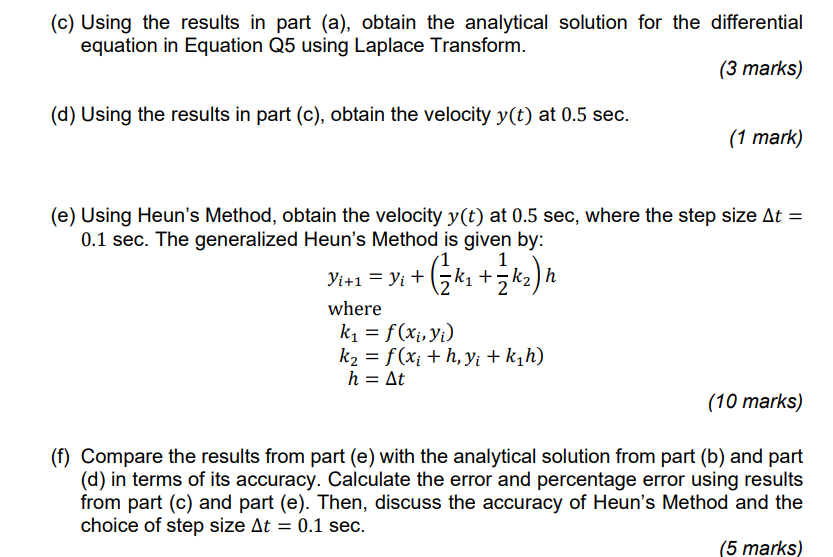Home / Expert Answers / Mechanical Engineering / a-simple-cart-system-is-represented-by-the-following-differential-equation-pa965

# (Solved): A simple cart system is represented by the following differential equation: \ ...???????

A simple cart system is represented by the following differential equation: $m \frac{d y(t)}{d t}+b y(t)=u(t)$ Equation Q5 where $$y(t)$$ is the velocity, $$u(t)$$ is the input force, $$m$$ is the fixed mass of the cart and $$b$$ is a constant friction coefficient. The initial conditions are $$y(0)=0$$ and $$u(0)=0$$. Assume that the mass $$m=0.1$$ and friction coefficient $$b=1$$ and the input force $$u(t)$$ is a unit step from $$t \geq 0$$. (a) Obtain the transfer function for the system in Equation Q5. (3 marks) (b) Does the Final Value Theorem apply to this system? Explain your reasoning. If yes, find the final value. (3 marks) (c) Using the results in part (a), obtain the analytical solution for the differential equation in Equation Q5 using Laplace Transform. (3 marks) (d) Using the results in part (c), obtain the velocity $$y(t)$$ at $$0.5 \mathrm{sec}$$. (1 mark) (e) Using Heun's Method, obtain the velocity $$y(t)$$ at $$0.5 \mathrm{sec}$$, where the step size $$\Delta t=$$ $$0.1 \mathrm{sec}$$. The generalized Heun's Method is given by: $\begin{array}{l} y_{i+1}=y_{i}+\left(\frac{1}{2} k_{1}+\frac{1}{2} k_{2}\right) h \\ \text { where } \\ k_{1}=f\left(x_{i}, y_{i}\right) \\ k_{2}=f\left(x_{i}+h, y_{i}+k_{1} h\right) \\ h=\Delta t \end{array}$ (10 marks) (f) Compare the results from part (e) with the analytical solution from part (b) and part (d) in terms of its accuracy. Calculate the error and percentage error using results from part (c) and part (e). Then, discuss the accuracy of Heun's Method and the choice of step size $$\Delta t=0.1 \mathrm{sec}$$.

We have an Answer from Expert